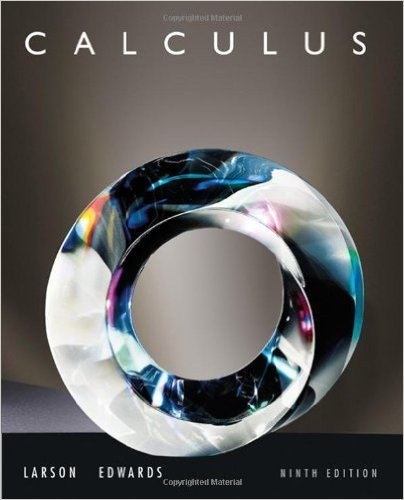×
×

# Solutions for Chapter 9.6: The Ratio and Root Tests## Full solutions for Calculus | 9th Edition

ISBN: 9780547167022Solutions for Chapter 9.6: The Ratio and Root Tests

Solutions for Chapter 9.6
4 5 0 396 Reviews
28
2
##### ISBN: 9780547167022

This expansive textbook survival guide covers the following chapters and their solutions. Chapter 9.6: The Ratio and Root Tests includes 111 full step-by-step solutions. Calculus was written by and is associated to the ISBN: 9780547167022. Since 111 problems in chapter 9.6: The Ratio and Root Tests have been answered, more than 61103 students have viewed full step-by-step solutions from this chapter. This textbook survival guide was created for the textbook: Calculus , edition: 9.

Key Calculus Terms and definitions covered in this textbook
• Absolute minimum

A value ƒ(c) is an absolute minimum value of ƒ if ƒ(c) ? ƒ(x)for all x in the domain of ƒ.

• Binomial theorem

A theorem that gives an expansion formula for (a + b)n

• Bounded above

A function is bounded above if there is a number B such that ƒ(x) ? B for all x in the domain of ƒ.

• Cofunction identity

An identity that relates the sine, secant, or tangent to the cosine, cosecant, or cotangent, respectively

• Identity matrix

A square matrix with 1’s in the main diagonal and 0’s elsewhere, p. 534.

• Identity properties

a + 0 = a, a ? 1 = a

• Infinite sequence

A function whose domain is the set of all natural numbers.

• Jump discontinuity at x a

limx:a - ƒ1x2 and limx:a + ƒ1x2 exist but are not equal

• Linear combination of vectors u and v

An expression au + bv , where a and b are real numbers

• Linear factorization theorem

A polynomial ƒ(x) of degree n > 0 has the factorization ƒ(x) = a(x1 - z1) 1x - i z 22 Á 1x - z n where the z1 are the zeros of ƒ

• Linear regression equation

Equation of a linear regression line

• Multiplication principle of probability

If A and B are independent events, then P(A and B) = P(A) # P(B). If Adepends on B, then P(A and B) = P(A|B) # P(B)

• Natural logarithm

A logarithm with base e.

• Parametric equations

Equations of the form x = ƒ(t) and y = g(t) for all t in an interval I. The variable t is the parameter and I is the parameter interval.

• Present value of an annuity T

he net amount of your money put into an annuity.

The formula x = -b 2b2 - 4ac2a used to solve ax 2 + bx + c = 0.

• Quartic function

A degree 4 polynomial function.

• Reflection through the origin

x, y and (-x,-y) are reflections of each other through the origin.

• Variation

See Power function.

• Vertex of a parabola

The point of intersection of a parabola and its line of symmetry.

×